The distribution of a (test) statistic T(yrep), or a pair of (test) statistics, over the simulated datasets in yrep, compared to the observed value T(y) computed from the data y. See the Plot Descriptions and Details sections, below, as well as Gabry et al. (2019).

ppc_stat(y, yrep, stat = "mean", ..., binwidth = NULL, breaks = NULL,
freq = TRUE)

ppc_stat_grouped(y, yrep, group, stat = "mean", ...,
facet_args = list(), binwidth = NULL, breaks = NULL, freq = TRUE)

ppc_stat_freqpoly_grouped(y, yrep, group, stat = "mean", ...,
facet_args = list(), binwidth = NULL, freq = TRUE)

ppc_stat_2d(y, yrep, stat = c("mean", "sd"), ..., size = 2.5,
alpha = 0.7)

## Arguments

y A vector of observations. See Details. An $$S$$ by $$N$$ matrix of draws from the posterior predictive distribution, where $$S$$ is the size of the posterior sample (or subset of the posterior sample used to generate yrep) and $$N$$ is the number of observations (the length of y). The columns of yrep should be in the same order as the data points in y for the plots to make sense. See Details for additional instructions. A single function or a string naming a function, except for ppc_stat_2d() which requires a vector of exactly two functions or function names. In all cases the function(s) should take a vector input and return a scalar statistic. If specified as a string (or strings) then the legend will display function names. If specified as a function (or functions) then generic naming is used in the legend. Currently unused. Passed to ggplot2::geom_histogram() to override the default binwidth. Passed to ggplot2::geom_histogram() as an alternative to binwidth. For histograms, freq=TRUE (the default) puts count on the y-axis. Setting freq=FALSE puts density on the y-axis. (For many plots the y-axis text is off by default. To view the count or density labels on the y-axis see the yaxis_text() convenience function.) A grouping variable (a vector or factor) the same length as y. Each value in group is interpreted as the group level pertaining to the corresponding value of y. A named list of arguments (other than facets) passed to ggplot2::facet_wrap() or ggplot2::facet_grid() to control faceting. Arguments passed to ggplot2::geom_point() to control the appearance of scatterplot points.

## Value

A ggplot object that can be further customized using the ggplot2 package.

## Details

For Binomial data, the plots will typically be most useful if y and yrep contain the "success" proportions (not discrete "success" or "failure" counts).

## Plot Descriptions

ppc_stat()

A histogram of the distribution of a test statistic computed by applying stat to each dataset (row) in yrep. The value of the statistic in the observed data, stat(y), is overlaid as a vertical line. More details on ppc_stat() can be found in Gabry et al. (2019).

ppc_stat_grouped(),ppc_stat_freqpoly_grouped()

The same as ppc_stat(), but a separate plot is generated for each level of a grouping variable. In the case of ppc_stat_freqpoly_grouped() the plots are frequency polygons rather than histograms. More details on ppc_stat_grouped() can be found in Gabry et al. (2019).

ppc_stat_2d()

A scatterplot showing the joint distribution of two test statistics computed over the datasets (rows) in yrep. The value of the statistics in the observed data is overlaid as large point.

Gabry, J. , Simpson, D. , Vehtari, A. , Betancourt, M. and Gelman, A. (2019), Visualization in Bayesian workflow. J. R. Stat. Soc. A, 182: 389-402. doi:10.1111/rssa.12378. (journal version, arXiv preprint, code on GitHub)

Gelman, A., Carlin, J. B., Stern, H. S., Dunson, D. B., Vehtari, A., and Rubin, D. B. (2013). Bayesian Data Analysis. Chapman & Hall/CRC Press, London, third edition. (Ch. 6)

Other PPCs: PPC-discrete, PPC-distributions, PPC-errors, PPC-intervals, PPC-loo, PPC-overview, PPC-scatterplots

## Examples

y <- example_y_data()
yrep <- example_yrep_draws()
ppc_stat(y, yrep)#> stat_bin() using bins = 30. Pick better value with binwidth.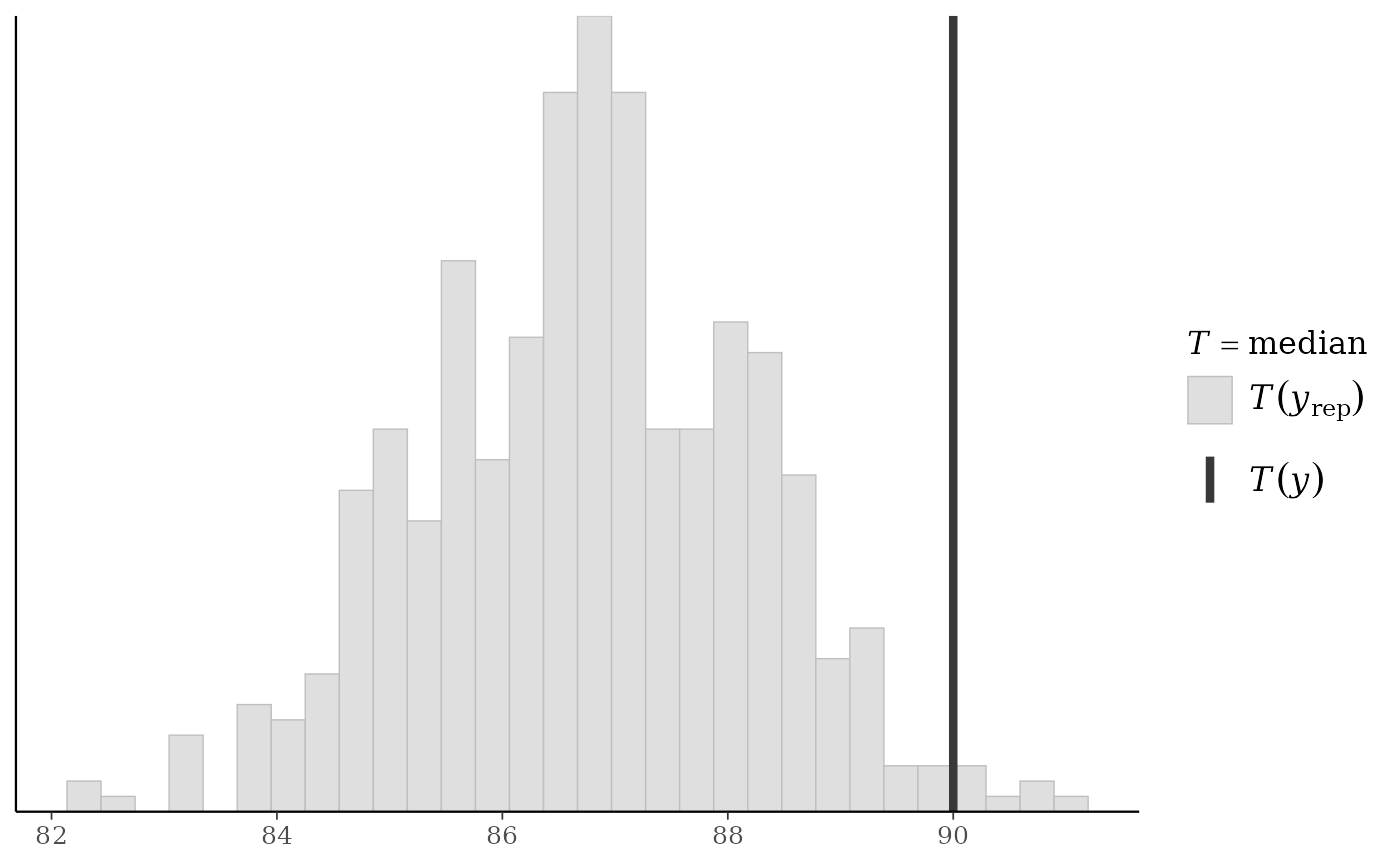ppc_stat(y, yrep, stat = "sd") + legend_none()#> stat_bin() using bins = 30. Pick better value with binwidth.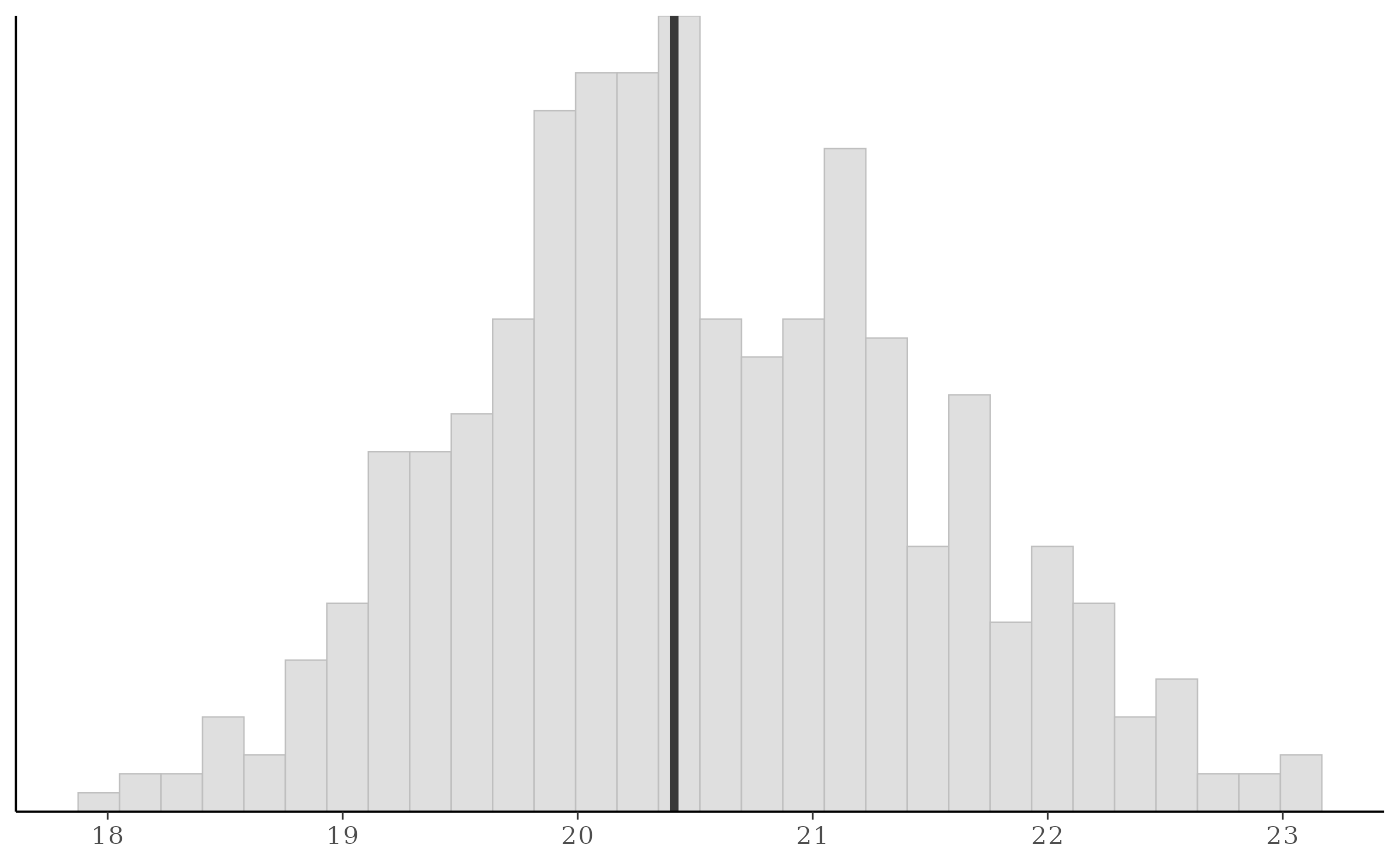ppc_stat_2d(y, yrep)ppc_stat_2d(y, yrep, stat = c("median", "mean")) + legend_move("bottom")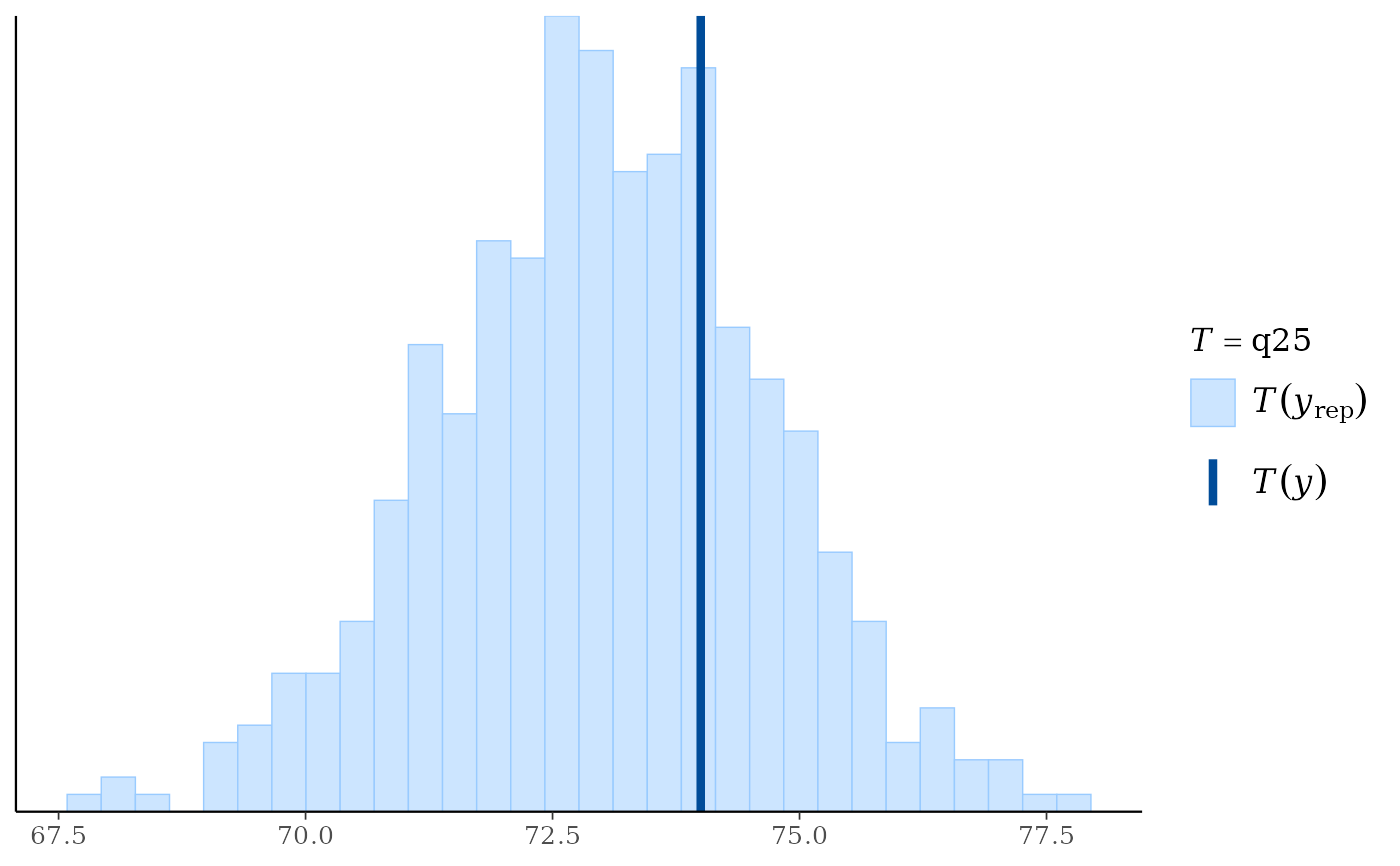color_scheme_set("teal")
group <- example_group_data()
ppc_stat_grouped(y, yrep, group)#> stat_bin() using bins = 30. Pick better value with binwidth.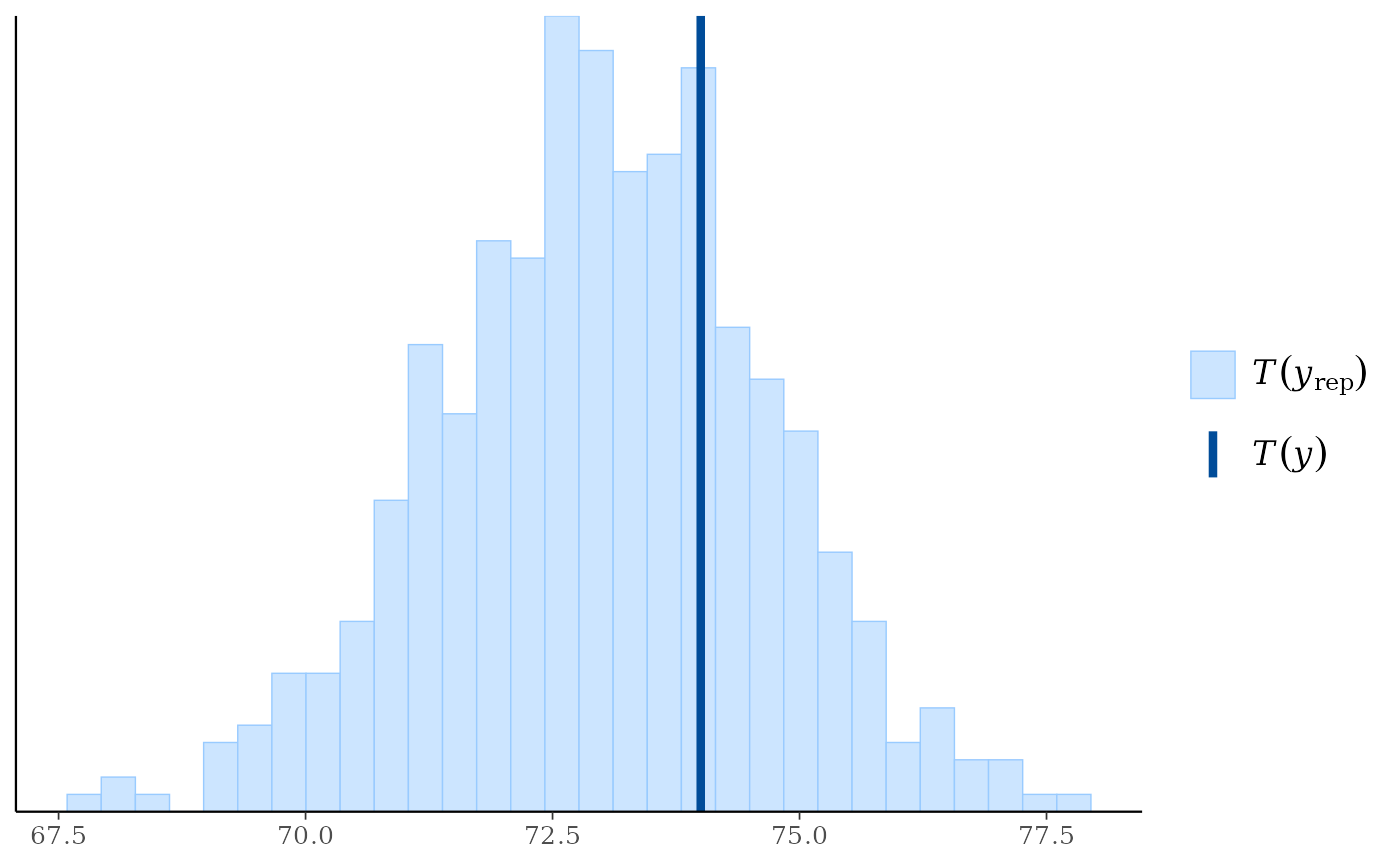color_scheme_set("mix-red-blue")
ppc_stat_freqpoly_grouped(y, yrep, group, facet_args = list(nrow = 2))#> stat_bin() using bins = 30. Pick better value with binwidth.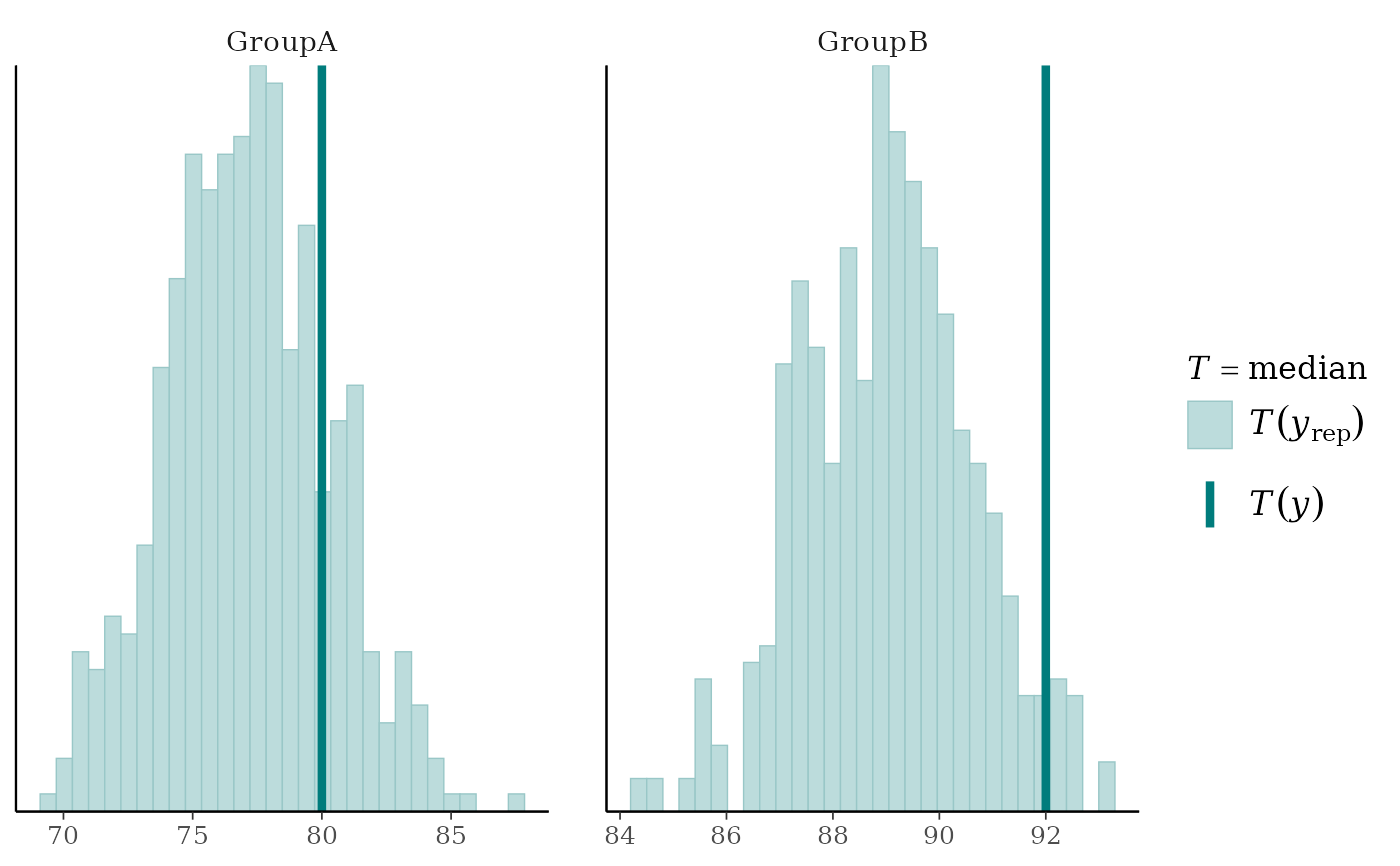# use your own function to compute test statistics
color_scheme_set("brightblue")
q25 <- function(y) quantile(y, 0.25)
ppc_stat(y, yrep, stat = "q25") # legend includes function name#> stat_bin() using bins = 30. Pick better value with binwidth.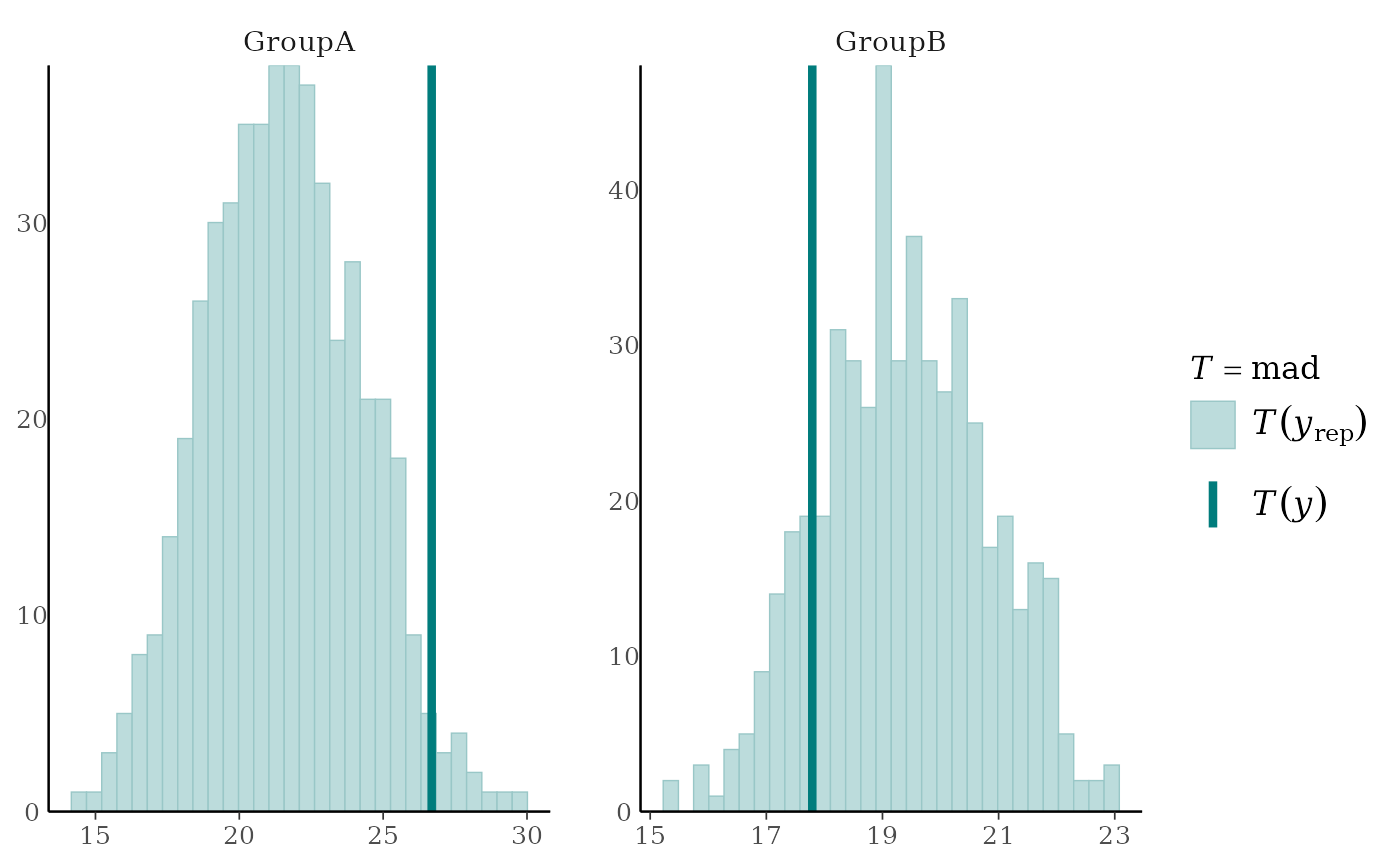# can define the function in the 'stat' argument but then
# the legend doesn't include a function name
ppc_stat(y, yrep, stat = function(y) quantile(y, 0.25))#> stat_bin() using bins = 30. Pick better value with binwidth.